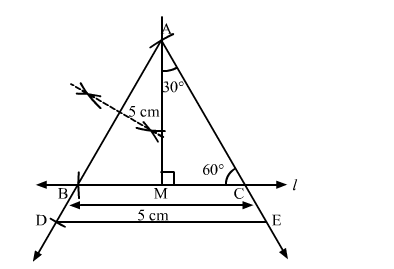# Construct a ∆ABC, in which BC = 5 cm, ∠C = 60° and altitude from A is equal to 3 cm.`
Question:

Construct a $\triangle A B C$, in which $B C=5 \mathrm{~cm}, \angle C=60^{\circ}$ and altitude from $A$ is equal to $3 \mathrm{~cm}$. Construct a $\triangle A D E$ similar to $\triangle A B C$, such that each side of $\triangle A D E$ is $\frac{3}{2}$ times the

corresponding side of ∆ABC. Write the steps of construction.

Solution:

Steps of Construction :

Step 1. Draw a line l .
Step 2. Draw an angle of 90o at M on l .
Step 3. Cut an arc of radius 3 cm on the perpendicular. Mark the point as A.

Step 4. With $\mathrm{A}$ as centre, make an angle of $30^{\circ}$ and let it cut $/$ at $\mathrm{C}$. We get $\angle A C B=60^{\circ}$.

Step 5. Cut an arc of 5 cm from C on l and mark the point as B.
Step 6. Join AB.
Thus, △ABC is obtained .

Step 7 . Extend $A B$ to $D$, such that $B D=\frac{1}{2} B C$.

Step 8. Draw $\mathrm{DE} \| B C$, cutting $\mathrm{AC}$ produced to $\mathrm{E}$.Then, $\triangle \mathrm{ADE}$ is the required triangle, each of whose sides is $\frac{3}{2}$ of the corresponding sides of $\triangle \mathrm{ABC}$.